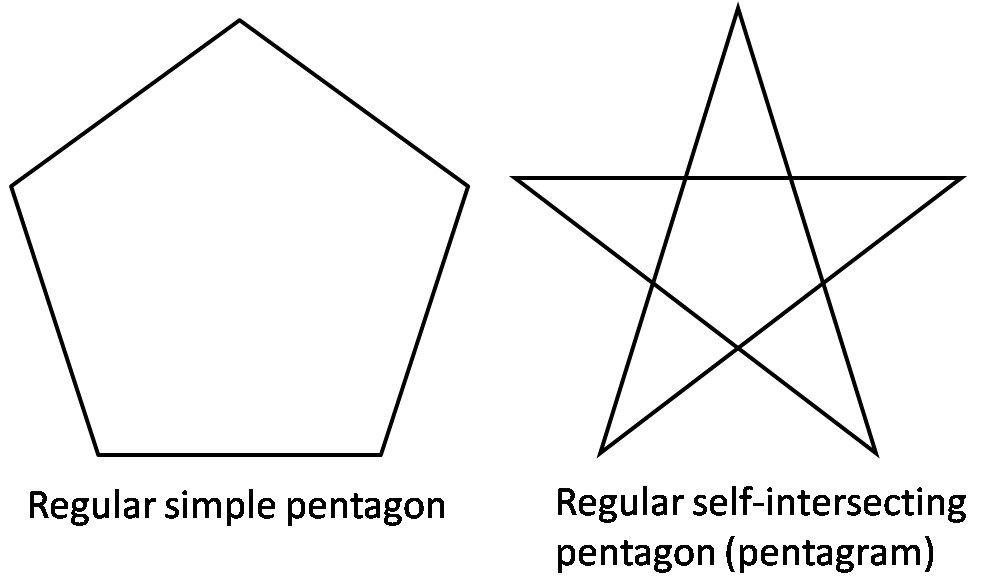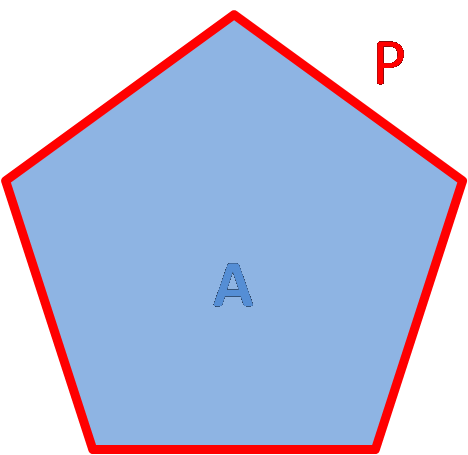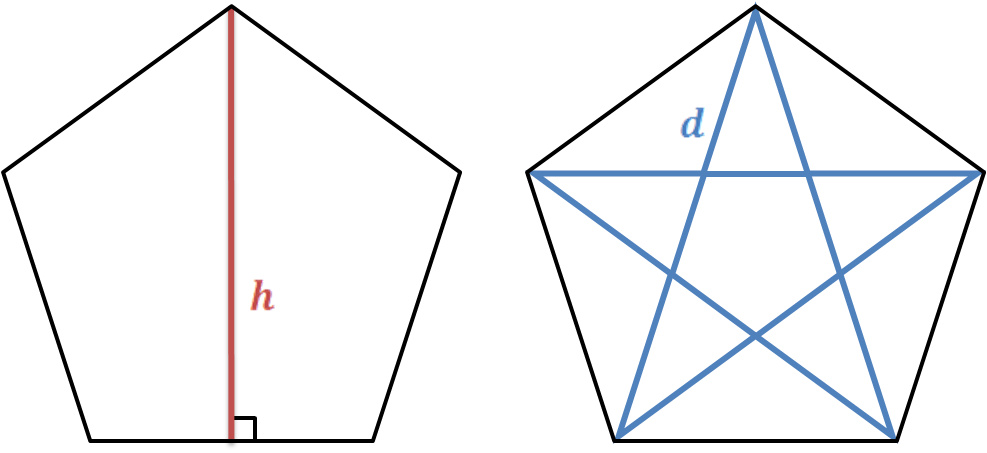# Pentagon Calculator

Created by Hanna Pamuła, PhD candidate
Reviewed by Bogna Szyk
Last updated: Dec 01, 2021

With this pentagon calculator, you'll find essential properties of a regular pentagon: side, diagonal, height, perimeter, and area, as well as the circumcircle and incircle radius. Type any value, and the remaining parameters will be calculated on the spot. If you are not sure what is a pentagon or how many sides does a pentagon have, keep scrolling and you'll find clarifying pictures with a short explanation.

## What is a pentagon? How many sides does a pentagon have?

Pentagon is a 5-sided polygon. Pentagon can be simple or self-intersecting.The sum of the internal angles in a simple pentagon is 540°, so every internal angle is equal to 108°. Regular simple pentagon has all five sides equal in length. (In this article, we use the term "regular pentagon" to describe a regular simple pentagon).

## Area and perimeter of a regular pentagon

Area A of a regular pentagon can be calculated from the formula:area = a² * √(25 + 10√5) / 4, where a is a side of a regular pentagon.

Also, you can find the area having the circumscribed circle radius:

area = 5 * R² * √[(5 + √5)/2] / 4, where R is an circumcircle radius.

Perimeter P of a regular pentagon is equal to side length multiplied by the number of vertices. Pentagon is a 5 sided polygon, so the perimeter is:

perimeter = 5 * a

## Height and diagonal of a regular pentagon

To calculate the height and diagonal of a regular pentagon, all you need to have given is the side length a:

diagonal = a * (1 + √5) / 2

height = a * √(5 + 2√5) / 2

Pentagon has five diagonals equal in length, which form a pentagram.## How to solve a regular pentagon using this pentagon calculator?Now as we know the pentagon definition, we can have a look at this step-by-step example:

1. Find out what is given. For a regular pentagon, one parameter is enough to find the remaining six.
2. Type the value into pentagon calculator. Let's take the most famous almost regular pentagon as an example - the Pentagon building, headquarters of the US Department of Defense. From the Wikipedia page we find out that it's 1414 ft wide - it's the height of the pentagram.The Pentagon, 1,414 feet, 431m (Light blue)

RMS Queen Mary 2, 1,132 feet, 345m (Pink)

US Navy's nuclear-powered USS Enterprise, 1,123 feet, 342m (Yellow)

Airship LZ 129 Hindenburg, 804 feet, 245m (Green)

Imperial Japanese Navy's Yamato, 863 feet, 263m (Dark blue)

Empire State Building, 1,454 feet, 443m (Grey)

Knock Nevis supertanker, 1,503 feet, 458m (Red)
Apple Park main building, 1,522 feet, 458m (Green)

1. The pentagon parameters appear! They are:
• side - 918.9 ft
• diagonal - 1486.8 ft
• perimeter - 4594 ft (0.87 mi)
• area - 33.35 ac
• circumcircle radius - 781.6 ft
• incircle radius - 632.4 ft

Did you notice how enormous it is? Have a look on the perimeter - it's almost a mile! In reality, each side of the building is ~921 feet long - looks like it's practically a regular pentagon!

## Other regular shapes

If you are interested in other regular shapes, have a look at our great tools:

Hanna Pamuła, PhD candidateSide (a)
in
Perimeter
in
Area
in²
Diagonal (d)
in
Height (h)
in
in
in
People also viewed…

### Helium balloons

Wondering how many helium balloons it would take to lift you up in the air? Try this helium balloons calculator! 🎈

### Podcasts

Do you feel like you could be doing something more productive or educational while on a bus? Or while cleaning the house? Well, why don't you dive into the rich world of podcasts! With this podcast calculator, we'll work out just how many great interviews or fascinating stories you can go through by reclaiming your 'dead time'!

### SSA triangle

Use our SSA triangle calculator to solve the ambiguous case of the law of sines.

### Special right triangles

What are the special right triangles formulas? How to solve special right triangles? Check out with this special right triangles calculator!